Rooted in statistical learning or Vapnik-Chervonenkis (VC) theory, (SVMs) are well positioned to generalize on yet-to-be-seen data. The SVM concepts presented in Chapter 3 can be generalized to become applicable to regression problems. As in classification, support vector regression (SVR) is characterized by the use of kernels, sparse solution, and VC control of the margin and the number of support vectors. Although less popular than SVM, SVR has been proven to be an effective tool in real-value function estimation. As a supervised-learning approach, SVR trains using a symmetrical loss function, which equally penalizes high and low misestimates. Using Vapnik’s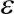-insensitive approach, a flexible tube of minimal radius is formed symmetrically around the estimated function, such that the absolute values of errors less than a certain thresholdare ignored both above and below the estimate. In this manner, points outside the tube are penalized, but those within the tube, either above or below the function, receive no penalty. One of the main advantages of SVR is that its computational complexity does not depend on the dimensionality of the input space. Additionally, it has excellent generalization capability, with high prediction accuracy.

This chapter is designed to provide an overview of SVR and Bayesian regression. It also presents a case study of a modified SVR applicable to circumstances in which it is critically necessary to eliminate or strictly limit underestimating a function.

## SVR Overview

The regression problem is a generalization of the classification problem, in which the model returns a continuous-valued output, as opposed to an output from a finite set. In other words, a regression model estimates a continuous-valued multivariate function.

SVMs solve binary classification problems by formulating them as convex optimization problems (Vapnik 1998). The optimization problem entails finding the maximum margin separating the hyperplane, while correctly classifying as many training points as possible. SVMs represent this optimal hyperplane with support vectors. The sparse solution and good generalization of the SVM lend themselves to adaptation to regression problems. SVM generalization to SVR is accomplished by introducing an ε-insensitive region around the function, called the ε-tube. This tube reformulates the optimization problem to find the tube that best approximates the continuous-valued function, while balancing model complexity and prediction error. More specifically, SVR is formulated as an optimization problem by first defining a convex ε-insensitive loss function to be minimized and finding the flattest tube that contains most of the training instances. Hence, a multiobjective function is constructed from the loss function and the geometrical properties of the tube. Then, the convex optimization, which has a unique solution, is solved, using appropriate numerical optimization algorithms. The hyperplane is represented in terms of support vectors, which are training samples that lie outside the boundary of the tube. As in SVM, the support vectors in SVR are the most influential instances that affect the shape of the tube, and the training and test data are assumed to be independent and identically distributed (iid), drawn from the same fixed but unknown probability distribution function in a supervised-learning context.

## SVR: Concepts, Mathematical Model, and Graphical Representation

SVR problem formulation is often best derived from a geometrical perspective, using the one-dimensional example in Figure 4-1. The continuous-valued function being approximated can be written as in Equation 4-1. For multidimensional data, you augment x by one and include b in the w vector to simply the mathematical notation, and obtain the multivariate regression in Equation 4-2.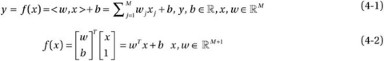SVR formulates this function approximation problem as an optimization problem that attempts to find the narrowest tube centered around the surface, while minimizing the prediction error, that is, the distance between the predicted and the desired outputs. The former condition produces the objective function in Equation 4-3, where ||w|| is the magnitude of the normal vector to the surface that is being approximated: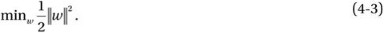To visualize how the magnitude of the weights can be interpreted as a measure of flatness, consider the following example: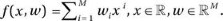Here, M is the order of the polynomial used to approximate a function. As the magnitude of the vector w increases, a greater number of w i are nonzero, resulting in higher-order solutions, as shown in Figure 4-2. The horizontal line is a 0th-order polynomial solution and has a very large deviation from the desired outputs, and thus, a large error. The linear function, a 1st-order polynomial, produces better approximations for a portion of the data but still underfits the training data. The 6th-order solution produces the best tradeoff between function flatness and prediction error. The highest-order solution has zero error but a high complexity and will most likely overfit the solution on yet to be seen data. The magnitude of w acts as a regularizing term and provides optimization problem control over the flatness of the solution.

The constraint is to minimize the error between the predicted value of the function for a given input and the actual output. SVR adopts an ε-insensitive loss function, penalizing predictions that are farther than ε from the desired output. The value of ε determines the width of the tube; a smaller value indicates a lower tolerance for error and also affects the number of support vectors and, consequently, the solution sparsity. Intuitively, the latter can be visualized for Figure 4-1. If ε is decreased, the boundary of the tube is shifted inward. Therefore, more datapoints are around the boundary, which indicates more support vectors. Similarly, increasing ε will result in fewer points around the boundary.

Because it is less sensitive to noisy inputs, the ε-insensitive region makes the model more robust. Several loss functions can be adopted, including the linear, quadratic, and Huber ε, as shown in Equations 4-4, 4-5, and 4-6, respectively. As demonstrated in Figure 4-3, the Huber loss function is smoother than the linear and quadratic functions, but it penalizes all deviations from the desired output, with greater penalty as the error increases. The choice of loss function is influenced by a priori information about the noise distribution affecting the data samples (Huber 1964), the model sparsity sought, and the training computational complexity. The loss functions presented here are symmetrical and convex. Although asymmetrical loss functions can be adopted to limit either underestimation or overestimation, the loss functions should be convex to ensure that the optimization problem has a unique solution that can be found in a finite number of steps. Throughout this chapter, the derivations will be based on the linear loss function of Equation 4-4.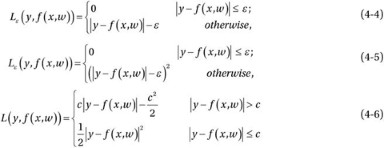### Asymmetrical Loss Functions

Some researchers have proposed modification to loss functions to make them asymmetrical. Shim, Yong, and Hwang (2011) used an asymmetrical ε-insensitive loss function in support vector quantile regression (SVQR) in an attempt to decrease the number of support vectors. The authors altered the insensitivity according to the quantile and achieved a sparser model. Schabe (1991) proposed a two-sided quadratic loss function and a quasi-quadratic s-loss function for Bayes parameter estimation, and Norstrom (1996) replaced the quadratic loss function with an asymmetrical loss function to derive a general class of functions that approach infinity near the origin for Bayesian risk analysis. Nath and Bhattacharyya (2007) presented a maximum margin classifier that bounds misclassification for each class differently, thus allowing for different tolerances levels. Lee, Hsieh, and Wang (2005) reformulated the typical SVR approach into a nonconstrained problem, thereby only solving a system of linear equations rather than a convex quadratic one. Pan and Pan (2006) compared three* different loss functions for economic tolerance design: Taguchi’s quadratic loss function, inverted normal loss function, and revised inverted normal loss function.

Adopting a soft-margin approach similar to that employed in SVM, slack variables ξ, ξ* can be added to guard against outliers. These variables determine how many points can be tolerated outside the tube illustrated in Figure 4-1.

Based on Equations 4-3 and 4-4, the optimization problem in Equation 4-7 is obtained; C is a regularization—thus, a tuneable parameter that gives more weight to minimizing the flatness, or the error, for this multiobjective optimization problem. For example, a larger C gives more weight to minimizing the error. This constrained quadratic optimization problem can be solved by finding the Lagrangian (see Equation 4-8). The Lagrange multipliers, or dual variables, are λ, λ*, α, α* and are nonnegative real numbers.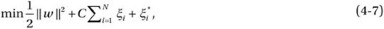subject to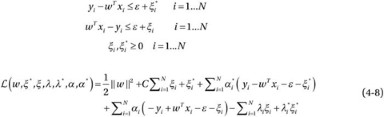The minimum of Equation 4-8 is found by taking its partial derivatives with respect to the variables and setting them equal to zero, based on the Karush-Kuhn-Tucker (KKT) conditions. The partial derivatives with respect to the Lagrange multipliers return the constraints, which have to be less than or equal to zero, as illustrated in Equation 4-9. The final KKT condition states that the product of the Lagrange multipliers and the constraints is equal to zero (see Equation 4-10). The Lagrange multipliers that are equal to zero correspond to data inside the tube, whereas the support vectors have nonzero-valued Lagrange multipliers. The solution is written in terms of the support vector only—hence, the solution sparsity. The function approximation is represented in Equation 4-12. By replacing Equation 4-9 in Equation 4-8, the dual form of the optimization problem can be written as shown in Equation 4-13.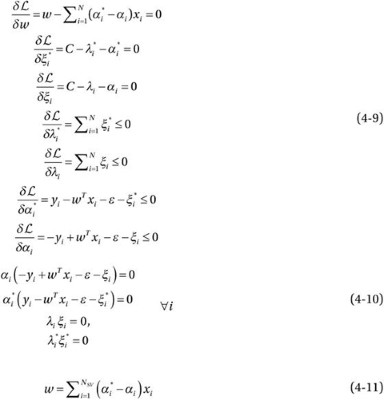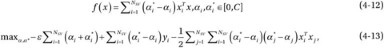subject to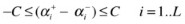At the beginning of this section, the weights vector w was augmented with the scalar b, and the derivation of the SVR’s mathematical formulation was carried out, disregarding the explicit computation of b (see Equation 4-2). However, b could have been calculated from the KKT conditions, as shown next.

Training data that belong to the outside of the boundary of the tube will have nonzero α i or α * i; they cannot both be zero, because that would mean that the instance (x i,y i) belongs to the lower and upper boundary, which is not possible. Therefore, the corresponding constraints will be satisfied with equality, as demonstrated in Equation 4-14. Furthermore, because the point is not outside the tube,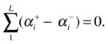, leading to the result in Equation 4-15 when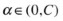. Equation 4-16 computes b. Performing the same analysis for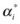, one gets Equations 4-17 and 4-18.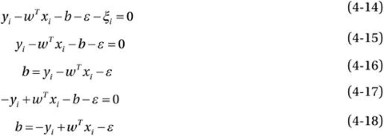Instead of using the KKT conditions, one could have also computed b, while solving the optimization problem, using the interior-point method, which can converge to an optimal solution in logarithmic time by navigating along the central path of the feasible region. The central path is determined by solving the primal and dual optimization problems simultaneously.

## Kernel SVR and Different Loss Functions: Mathematical Model and Graphical Representation

The previous section dealt with data in the feature space, assuming f(x) is linear. For non linear functions, the data can be mapped into a higher dimensional space, called kernel space, to achieve a higher accuracy, using kernels that satisfy Mercer’s condition (see Figure 4-4), as discussed previously for classification. Therefore, replacing all instances of x in Equations 4-1–4-18 with k(x i,x j) yields the primal formulation shown in Equation 4-19, where φ(.) is the transformation from feature to kernel space. Equation 4-20 describes the new weight vector in terms of the transformed input. The dual problem is represented in Equation 4-21, and the function approximation f(x) is in Equation 4-22, where k(.,.), the kernel, is as illustrated in Equation 4-23.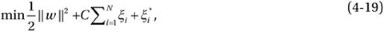subject to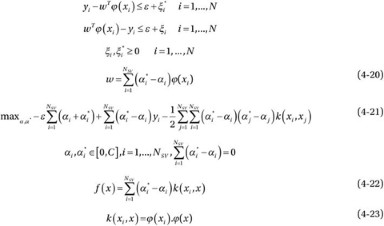## Bayesian Linear Regression

Unlike SVR, Bayesian linear regression is a generative, as opposed to discriminant, method, that builds linear regression models based on Bayesian inference. After specifying a model, the method computes the posterior distribution of parameters and model predictions. This statistical analysis allows the method to determine model complexity during training, which results in a model that is less likely to overfit.

For simplicity, assume that a single output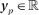are predicted using the model parameters w learned from a set of predictor variables X sized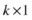and observations Y sized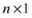. The observations Y are assumed to have the distribution in Equation 4-24, where σ 2 is the variance of the uncertainty in the observations: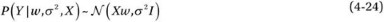Once the model has been specified, the model parameters’ posterior distributions can be estimated. This is done by first assuming a prior distribution of the model parameters (see Equation 4-25). Given the model variance and observations, the posterior distribution of the model parameters (which is Gaussian) is as shown in Equation 4-26, with the mean computed in Equation 4-27, and the standard deviation scale factor, in Equation 4-28. The mean is simply the Moore-Penrose pseudoinverse of the predictive variables multiplied by the observations. Given some observations, the posterior probability of the model variance is computed, and an inverse chi-squared distribution (see Equation 4-29), with n – k degrees of freedom and a scale factor s 2 (see Equation 4-30), is obtained. The scale factor is the error between the model’s predicted output and an observation.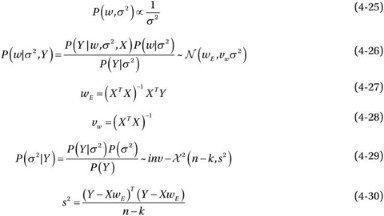The marginal posterior distribution of the model parameters, given the observations, is a multivariate Student’s t-distribution, shown in Equation 4-31 and computed in Equation 4-32, with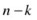degrees of freedom, w E mean, and s 2 scale factor, as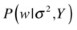has a normal distribution, and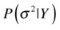has an inverse chi-squared distribution.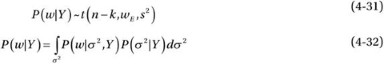Given the model parameter probability distributions and a set of predictive variables X p, the marginal posterior predictive distribution Y p, which is a multivariate Student’s t-distribution (see Equation 4-33) can be determined. The mean is computed in Equation 4-34, and the variance, in Equation 4-35. The predictive distribution variance depends on the uncertainty in the observed data and the model parameters.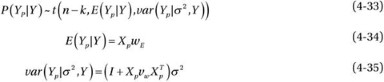The concept of Bayesian regression is displayed in Figure 4-5, in which the sample input data available during training would have been generated by a Gaussian distribution. If these instances represent their population well, the regression model is expected to generalize well.

### Discriminant vs. Generative Models

A generative approach models the joint probability distribution of the data and class labels p(x, C k), based on the prior probability distributions of the class labels p(C k) and the likelihood probability distribution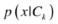. The joint distribution computes the posterior probability distributions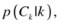which will be used to map datapoints to class labels.

A discriminant approach directly computes the posterior probability distributions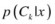without computing the joint probability distribution p(x,C k). A discriminant approach produces a mapping from the datapoints to the class labels without computing probability distributions. Therefore, this approach performs the inference and decision stages in one step.

Generative

• Robust to outliers

• Can easily update decision model

• Allows combination of classifiers trained on different types of data by applying probability rules

• Can improve prediction accuracy by measuring confidence in classification based on posterior distributions and not making predictions when confidence is low

• Computationally demanding

• Requires a lot of training data

• Suffers from the curse of dimensionality

Discriminant

• Computationally less demanding

• Simple to implement

• Sensitive to noisy data and outliers

• Requires retraining for any changes in the decision model

## Asymmetrical SVR for Power Prediction: Case Study

Justification: In many instances of approximation, there is an uneven consequence of misprediction, based on whether the error is above or below the target value (Stockman et al. 2012a, 2012b). For example, in power prediction an incorrect low estimate may be of much more concern than an overestimate. Underpredicting can lead to insufficient cooling of datacenters, inadequate uninterruptible power supply (UPS), unavailable processor resources, needless powering down of chip components, and so on. In the case of forest fire behavior prediction, a lower estimate of the threat can lead to greater property damage as well as loss of life, owing to a lack of adequate supply of personnel and equipment.

In these instances, it is crucial to minimize misestimates on one side of a boundary, even at the risk of reducing the accuracy of the entire estimation. It is necessary to restrict the loss function so that a minimal number of under- or overestimates occur. This leads to an asymmetrical loss function for training, in which a greater penalty is applied when the misestimate is on the wrong side of the boundary.

Approach: Asymmetrical and lower-bounded SVR (ALB-SVR) was proposed by Stockman, Awad, and Khanna (2012a). This approach modifies the SVR loss functions and corresponding error functions, such that the ε-tube is only above the function, as demonstrated in Figure 4-6. The penalty parameter C is split into C+ and C- so that different penalties can be applied to the upper and lower mispredictions.

ALB-SVR uses the Huber insensitive loss function (Popov and Sautin 2008). This function is similar to the ε-insensitive loss function; however, it increases quadratically for small errors outside the ε-bound but below a certain threshold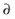> ε and then linearly beyond. This makes it robust with respect to outliers. The Huber insensitive loss function is represented by: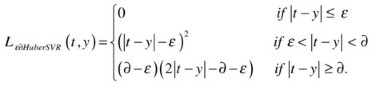ALB-SVR modifies the Huber insensitive loss function as follows: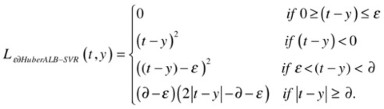Thus, the solution is: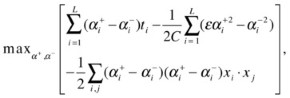and the resulting optimization problem: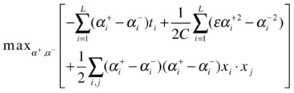By substituting the new loss function, ALB-SVR’s empirical risk becomes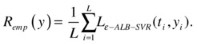The maximum additional empirical risk for ALB-SVR can be computed as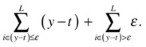Validation: ALB-SVR was tested on a dataset used by David et al. (2010) and Stockman et al. (2010) that consists of 17,765 samples of five attributes of memory activity counters, with the actual corresponding power consumed in watts, as measured directly by a memory power riser. The memory power model attributes are activity, read, write, CKE = high, and CKE = low. ALB-SVR was implemented with a modified version of LIBSVM (Chang and Lin 2011) for ALB-SVR. Simulation results (see Figures 4-74-9) took the average of ten runs of threefold cross-validation of a radial basis function (RBF) kernel, with a combination of grid search and heuristic experimentation to find the best metaparameters ε, g, C +, and C .

In SVR, support vectors are those points that lie outside the ε-tube. The smaller the value of ε, the more points that lie outside the tube and hence the greater the number of support vectors. With ALB-SVR the -tube is cut in half, and the lower -bound is dropped. Therefore, for the same g and parameters, more points lie outside the tube, and there are a larger number of support vectors. This means that the number of support vectors is greater for ALB-SVR than for SVR. This increase in the number of support vectors indicates that using ALB-SVR has some negative effects on the complexity of the estimating function. Although the percentage relative error data set was higher (5.06 percent), this is acceptable, because the main purpose was to reduce the number of underestimates and this was achieved.

## References

Chang, Chih-Chung, and Chih-Jen Lin. “LIBSVM: A Library for Support Vector Machines,” in “Large-Scale Machine Learning,” edited by C. Ling, special issue, ACM Transactions on Intelligent Systems and Technology 2, no. 3 (2011). www.csie.ntu.edu.tw/∼cjlin/papers/libsvm.pdf.

David, Howard, Eugene Gorbatov, Ulf R. Hanebutte, Rahul Khanna, and Christian Le. “RAPL: Memory Power Estimation and Capping.” In Proceedings of the 2010 ACM/IEEE International Symposium on Low-Power Electronics and Design (ISLPED), August 18–20, 2010, Austin, TX, 189–194. Piscataway, NJ: Institute for Electrical and Electronics Engineers, 2010.

Huber, Peter J. “Robust Estimation of a Location Parameter.” Annals of Mathematical Statistics 35, no. 1 (1964): 73–101.

Lee, Yuh-Jye, Wen-Feng Hsieh, and Chien-Ming Huang. “ε-SSVR: A Smooth Support Vector Machine for ε-Insensitive Regression.” IEEE Transactions on Knowledge and Data Engineering 17, no. 5 (2005): 678–685.

Nath, J. Saketha, and Chiranjib Bhattacharyya. “Maximum Margin Classifiers with Specified False Positive and False Negative Error Rates.” In Proceedings of the Seventh SIAM International Conference on Data Mining, April 26–28, 2007, Minneapolis, MN, 35–46. 2007. http://dblp.uni-trier.de/rec/bibtex/conf/sdm/NathB07 .

Norstrom, Jan Gerhard. “The Use of Precautionary Loss Functions in Risk Analysis.” IEEE Transactions on Reliability 45, no. 3 (1996): 400–403.

Pan, Jeh-Nan, and Jianbiao Pan. “A Comparative Study of Various Loss Functions in the Economic Tolerance Design.” In Proceedings of the 2006 IEEE International Conference on Management of Innovation and Technology, June 21–23, 2006, Singapore, China, 783–787. Piscataway, NJ: Institute of Electrical and Electronics Engineers, 2006.

Popov, A. A, and A. S. Sautin. “Loss Functions Analysis in Support Vector Regression,” 9th International Conference on Actual Problems of Electronic Instrument Engineering, September 23–25, 2008, Novosibirsk, Russia, 198. Piscataway, NJ: Institute of Electrical and Electronics Engineers, 2008.

Schabe, H. “Bayes Estimates Under Asymmetric Loss.” IEEE Transactions on Reliability 40, no. 1 (1991): 63–67.

Shim, Joo Yong, and Chang Ha Hwang. “Support Vector Quantile Regression Using Asymmetric ε-Insensitive Loss Function.” Communications for Statistical Applications and Methods 18, no. 2 (2011): 165–170.

Stockman, Melissa, Mariette Awad, and Rahul Khanna. “Asymmetrical and Lower Bounded Support Vector Regression for Power Prediction.” Intel Technology Journal 16, no. 2 (2012a).

Stockman, Melissa, Mariette Awad, Rahul Khanna, Christian Le, Howard David, Eugene Gorbatov, and Ulf R. Hanebutte. “A Novel Approach to Memory Power Estimation Using Machine Learning.” In Proceedings of the 2010 International Conference on Energy Aware Computing (ICEAC), December 16–18, 2010, Cairo, Egypt, 1–3. Piscataway, NJ: Institute for Electrical and Electronics Engineers, 2010.

Stockman, Melissa, Randa S. El Ramli, Mariette Awad, and Rabih Jabr. “An Asymmetrical and Quadratic Support Vector Regression Loss Function for Beirut Short Term Load Forecast.” In 2012 IEEE International Conference on Systems, Man, and Cybernetics (SMC), October 14–17, 2012, Seoul, Korea, 651–656. Piscataway, NJ: Institute of Electrical and Electronics Engineers, 2012b.

Vapnik, Vladimir N. Statistical Learning Theory. New York: Wiley, 1998.